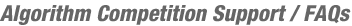JOINData Feeds

## Data Feeds

TopCoder provides access to some of it's data in the form of XML. Below you will find a listing of the available feeds. Please keep in mind that none of the feeds provide ordered data.

Coder List
This feed provides a list of coders who have competed in Algorithm, Development or Design competitions. It includes the following fields:

• user id
• handle
• country
• algorithm rating
• algorithm volatility
• number of rated algorithm events
• design rating
• design volatility
• number of rated design events
• development rating
• development volatility
• number of rated development events
FEED:
http://www.topcoder.com/tc?module=BasicData&c=dd_coder_list

Active Algorithm Coder List
This feed provides a list of coders who have been rated in an algorithm competition within the last 180 days. It includes the following fields:

• user id
• handle
• algorithm rating
• algorithm volatility
• number of rated algorithm events
FEED:
http://www.topcoder.com/tc?module=BasicData&c=dd_active_algorithm_list

Algorithm Round List
This feed provides a list of algorithm rounds.

• round id
• full name
• short name
• round type
• date
FEED:
http://www.topcoder.com/tc?module=BasicData&c=dd_round_list

Algorithm Round Results
This feed provides details about all the competitors in an algorithm round. It includes the following fields:

• room id
• room_name
• user id
• handle
• prize ("hidden" if the user has opted to hide his/her earnings)
• old rating
• new rating
• new volatility
• num ratings
• room placed
• division placed
• challengepoints
• system test points
• defense points
• submission points
• final points
• division
• problems presented
• problems submitted
• problems correct
• problems failed by system test
• problems failed by challenge
• problems opened
• problems left open
• rated flag (1 or 0)
• level one problem id
• level one submission points
• level one final points
• level one status
• level one time elapsed
• level one placed
• level one language
• level two problem id
• level two submission points
• level two final points
• level two status
• level two time elapsed
• level two placed
• level two language
• level three problem id
• level three submission points
• level three final points
• level three status
• level three time elapsed
• level three placed
• level three language
FEED:
http://www.topcoder.com/tc?module=BasicData&c=dd_round_results&rd=ROUND_ID

You will need to replace "ROUND_ID" in the query string to get data about a particular round.

Algorithm Rating History
This feed provides data about the algorithm rating history for a particular competitor. It includes the following fields:

• handle
• user id
• round id
• round name
• date
• old rating
• new rating
• volatility
• rank
• percentile
FEED:
http://www.topcoder.com/tc?module=BasicData&c=dd_rating_history&cr=USER_ID

You will need to replace "USER_ID" in the query string to get data about a particular competitor.